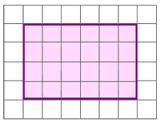# A box 4

A box open at the top has a rectangular base of 200mmx300mm and an altitude of 150mm. If the base and the sides are 10mm thick, find the total surface area of the box.

A =  3988 cm2

### Step-by-step explanation:Did you find an error or inaccuracy? Feel free to write us. Thank you!

Showing 1 comment:
Math student
Calculate the volume and total surface area in centimeters of a triangular prism if it has a rectangular base with a=4cm and hypotenuse c=50mm and height of the prism is 0.12dm.

Tips for related online calculators
Do you want to convert area units?

#### Grade of the word problem:

We encourage you to watch this tutorial video on this math problem: